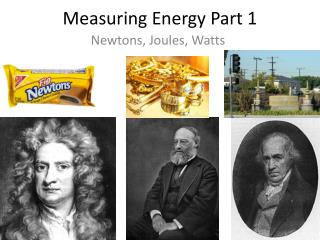Download PresentationMeasuring Energy Part 1

# Measuring Energy Part 1 - PowerPoint PPT PresentationDownload Presentation## Measuring Energy Part 1

- - - - - - - - - - - - - - - - - - - - - - - - - - - E N D - - - - - - - - - - - - - - - - - - - - - - - - - - -
##### Presentation Transcript

1. Measuring Energy Part 1 Newtons, Joules, Watts

2. Energy Use in Canada • Canadian households used 1,425 petajoules of energyin 2011(StatsCan) • 1 petajoule is approximately the amount of energy needed to run the TTC subway for one year

3. Newton (N) • SI unit of FORCE • Force is a push or pull against an object • 1 Newton is the amount of force needed to accelerate a mass of 1 kg by 1 meter/s2 N = kg x m/s2 A newton will move 1 kg of weight • one meter in the first second, • 2m in the second second • 3m in the third second

4. Newton (N) 1 KG 1 KG 1 KG 1 KG 1 KG 1 KG Time 1 Second 2 Seconds 3 Seconds

5. Joule (J) • SI unit of energy and work • Energy is the ability to do work • Work is the energy transferred when a force moves an object • 1 joule is the work done/energy transferred when 1 newton of force moves an object 1m. J = N x m (N=newton, m=meter) One joule equals the energy needed to lift an apple from the floor to the tabletop, about 1m.

6. Watt (W) • The SI unit of power • Power is the rate at which work is done • One watt is equal to 1 joule of energy produced per second W = J/s (j=joule, s=sec)

7. Watt hours (Wh) • Light bulbs are rated according to the power they use • this is measured in Watt hours (Wh). Since there are 3600 (60 x 60) seconds in an hour one watt hour = 3 600 joules of energy • A 20 watt lightbulb uses 20 watts of power in an hour. • A 20 watt bulb uses 3 600 X 20 = 72 000 joules of energy

8. Kilowatts (KW) • 1000 Watts is a kilowatt (KW) • A kilowatt hour (KWh) is equal to 1000 watt hours (Wh) One KWh = 1000 watt hours 1000 watt hours = 1000 x 3 600 = 3 600 000 joules